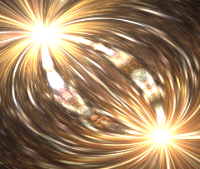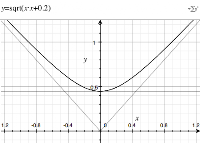## Monday, June 27, 2011

### CAD on OSX with Blender and Rhino - DWG

The majority of the engineering world use AutoCAD-like products for creating drawings, with typical exchange formats of DWG, DXF, SAT or IGES. (I wrote some tips on using AutoCAD and SolidEdge here) Unfortunately on OSX there has been historically little choice. I asked Stefan Boeykens who runs the CAD 3D blog for suggestions, and compiled a list of possibilities:
• AutoCAD have a Mac OSX version. Obviously 100% DWG support, the downside is the price (Retails for \$3,995, 30 day trial)
• iCADmac is a mac port of progeCAD, that is actually very good on OSX (still a poorer cousin of AutoCAD). I use this for 2D CAD work on OSX. (Retails ~\$1000, 30 day trial)
• ARES commander is a cross platform CAD package for Windows,OSX,Linux. So there should be no compatibility issues between. I've not tried it out myself. (Retails \$795, 30 day trial)
• Blender is a free cross platform 3D package, that does have DWG/DXF plugin support. Unfortunately, the DWG support is not very good, and it is difficult to get the plugin to work under OSX and Linux. (free, Open Source)
• Rhino 3D, a free trial version for OSX at the moment. (Retail expected ~\$1000, free unrestricted trial while in beta)
Although Stefan mentioned some issues with Rhino3D, it worked perfectly with all my CAD files, and it is still an alpha product at the moment. Rhino3D supports a lot of file formats to a high standard:
• 3dm (Rhino format)
• 3ds (3d Studio, original not Max)
• dwg (AutoCAD - I tested the 2007 version)
• fbx (MotionBuilder)
• iges (IGES)
• lwo (Lightwave)
• obj (Wavefront)
• sat (ACIS) [Export only]
• stl (Stereolithography)
• vrml (VRML, also wrl file format)
In the end, I only used Rhino3D to convert the data and used Blender for doing most of my data manipulation, as I already have a number of scripts for it (See Convex hull generation for Blender). Every time I get back to using Blender after a pause it takes me a while to get used to the interface, here are some common commands I use when manipulating objects to conform to a given coordinate system:
• space - add an object
• g - move (follow by x,y,z to select an axis)
• r - rotate (follow by x,y,z to select an axis)
• a - selects all objects
• h - hide objects
• . - zooms so you can see all
• 0 - switches to camera view (F9 - edit panel, you can set the FOV by altering 'D')
• n - displays the current transformation matrix (To find the global coordinates you should view the Object in edit mode)
• SHIFT+c - centres the cursor
• View->View properties - allows you to manually enter the cursor position
• Object->Transform->Center Cursor - allows you to re-define the center of the object
• CTRL+a - collapses the rotation/scale transformation matrices. (see above for setting the position)
• CTRL+p - to parent objects, allowing you to move them in groups
This Blender keyboard shortcut map always comes in handy too!

## Friday, June 17, 2011

### Deform (textured interference) effect in WebGL'deform' interference effect
This effect is one of my all time favourites. It is somewhat similar to the XOR bitplasma effect with which I started the tutorial series based on iq's shader toy. This effect is an 'interference' of two points based on the subtraction of the radii and angles.

Jumping straight to the solution; the code we are trying to decipher is:
``` float a1 = atan(p.y-offset1.y,p.x-offset1.x); float r1 = sqrt(dot(p-offset1,p-offset1)); float a2 = atan(p.y-offset2.y,p.x-offset2.x); float r2 = sqrt(dot(p-offset2,p-offset2)); vec2 uv; uv.x =(r1-r2); uv.y =(a1-a2);```
` `r1

So why does it look the way it looks?r2

Lets start with the radius part. If we just look at one radius (r1) we would expect to see a steadily increasing value radiating out from our offset point (in all my examples this will be at 0.3)

And this is exactly what we see when we plot this. If we plot the inverse (negative r2, offset to -0.3) we should see the inverse, that is, a steadily decreasing value radiating from the offset point.r1-r2

So if we combine these (r1-r2) we get a nice smooth surface transition between these two patterns. Where does this blending come from?

Lets take it back a step and look at our problem in just one dimension. Our definition of the radius is sqrt(x*x+y*y), in 1D we can plot x*x and sqrt(x*x):

Coming back to the generation of the surface, we can plot two interfering 1D functions, that is sqrt((x-0.3)*(x-0.3)) and sqrt((x+0.3)*(x+0.3)), and the difference (i.e. the first subtract the second). This gives us a ramped step between the functions as each reaches its turning point.

We can see the same feature in the surface, if we view it from an appropriate angle.r1-r2, alternative viewWe still haven't discovered the cause of the smooth blending that gives such a nice visual effect. When we add a constant (i.e. offset) to the function sqrt(x*x+k), notice how we now have the turning point at sqrt(k) and have a parabola instead. This is effectively evaluating sqrt(x*x+y*y) for some constant y=sqrt(k).

So, if we now consider the two extremes, again plotting in 1D but considering the 2D case for both our x-offsets of =-0.3 and 0.3 we get a set of blended curves (dark blue/red is y=-0.3, light blue/purple is y = 0.3).

And that is where our full surface comes from.Left: atan(x) Right: atan(1/y)
Now we want to understand the blend in the angles. The generating function is nothing more complex than atan(x/y). Below is a graph of atan(x), followed by atan(1/y). Note: There are different viewing angles for each function so it is easier to see the curvature.

If we combine these two equations into one (i.e. atan(x/y)) we get:atan(x/y)

Now, we just need to understand the interference pattern that is generated from two of these functions interacting. I'm going to take a shortcut here and just let an animated gif tell the story. This animation shows a discretized version of the angles (first in steps of ~60 degrees, then ~30, etc.) interacting for the equation a1-a2 from the code snippet.a1-a2 discretized and animated (open as a separate image)

From the gif you can see how the curves evolve. To understand these better we can take a look at how this curve pattern comes about for a constant difference angle. If we modify the end of our original shader code to:
``` uv.y = (a2-a1); float k = 0.0; if ((uv.y > 0.3) && (uv.y<0.31)) k = 1.0; gl_FragColor = vec4(col.r+k,col.g+k,col.b+k,1.0); ```

The resulting image is still the original effect, but one of the curves is highlighted (in white). This represents a curve where (a2-a1) ~= 0.3

We can understand this curve by generating a set of triangles in which two vertices are fixed, and the difference between two angles is held constant. By altering one angle the final vertex will generate the sweeping curve in the original image (See Law of Sines). In the example below, we vary a1 and hold a2 as (a1 - 30 degrees).

This generates our final explanatory image of the sweeping triangles:

Finally! The full effect has been deciphered, click the button below to see it in action. (You will need a WebGL enabled browser)

Your browser doesn't appear to support the HTML5 `<canvas>` element.

## Monday, June 13, 2011

### Demoparties

Australia is hosting another demo party, this time in Sydney called Flashback 2011. It will be interesting to see what gets released.

For a more international focus there is the Mozilla Demoparty with WebGL, CSS, GIF animation, and Audio competitions. The 'event' started on the 10th of June, and submissions close on the 30th of July. Take a look at the original announcement of the Mozilla Demoparty incase you want to organize a local contributing event.

If your looking for a modern tracker to make your audio you can try the open source Schism Tracker (Impulse Tracker clone). (Daniel Gagan has a nice blog post on various methods for implementing HTML5 audio loops) For your WebGL needs, there are a number of libraries/engines to try:
Should be enough to get you started. Of related interest is a description of Brandan Jones' WebGL renderer for id's Rage levels.

## Sunday, June 05, 2011

### Catchup Post: Robotics and Physical Simulations

A number of robotics related bits of interest from the last few months:
Finally Heat-1 the space rocket build by danish amateurs Copenhagen Suborbitals successfully launched. (TED talk here). See the video below.